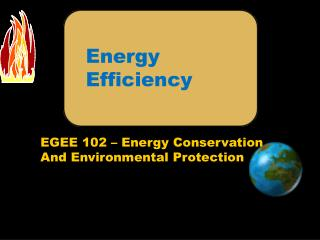DownloadDownload PresentationEnergy Efficiency

# Energy Efficiency

Télécharger la présentation## Energy Efficiency

- - - - - - - - - - - - - - - - - - - - - - - - - - - E N D - - - - - - - - - - - - - - - - - - - - - - - - - - -
##### Presentation Transcript

1. Energy Efficiency

2. Definition and Scope of Energy Efficiency • Energy efficiency improvements refer to a reduction in the energy used for a given service (heating, lighting, etc.) or level of activity. • The reduction in the energy consumption is usually associated with technological changes, but not always since it can also result from better organization and management or improved economic conditions in the sector ("non-technical factors").

3. Efficiency of Energy Conversion • If we are more efficient with the energy we already have there will be less pollution, less reliance on foreign oil and increased domestic security.

4. Energy Conversion Device Energy Efficiency Useful Energy Output Energy Input Energy Dissipated to the Surroundings

5. Illustration • An electric motor consumes 100 watts (a joule per second (J/s)) of power to obtain 90 watts of mechanical power. Determine its efficiency ? = 90 W x 100 = 90 % 100 W

6. Efficiency of Some Common Devices Device Electric Motor Home Oil Furnace Home Coal Furnace Steam Boiler (power plant) Power Plant (thermal) Automobile Engine Light Bulb-Fluorescent Efficiency 90 65 55 36 25 20 5

7. Vehicle Efficiency – Gasoline Engine 25% Of the gasoline is used to propel a car, the rest is “lost” as heat. i.e an efficiency of 0.25 Source: Energy Sources/Applications/Alternatives

8. Heat Engine • A heat engine is any device which converts heat energy into mechanical energy. • Accounts for 50% of our energy conversion devices

9. Carnot Efficiency • Maximum efficiency that can be obtained for a heat engine Temperature is in ° Kelvin !!!!!!!

10. Illustration For a coal-fired utility boiler, The temperature of high pressure steam would be about 540°C and T cold, the cooling tower water temperature would be about 20°C. Calculate the Carnot efficiency of the power plant ?

11. 540 ° C = 540 +273 ° K = 813 °K 20 °C = 20 + 273 = 293 °K

12. Inference A maximum of 64% of the fuel energy can go to generation. To make the Carnot efficiency as high as possible, either T hot should be increased or T cold should be decreased.

13. Schematic Diagram of a Power Plant

14. Boiler Components

15. Overall Efficiency Overall Eff = Electric Energy Output (BTU) x 100 Chemical Energy Input (BTU) = 35 BTU x 100 100 BTU = 35% Overall Efficiency of a series of devices = (Thermal Energy)x(Mechanical Energy) x(Electrical Energy) Chemical Energy Thermal Energy Mechanical Energy

16. Overall Efficiency Cont.. (Thermal Energy)x(Mechanical Energy) x(Electrical Energy) Chemical Energy Thermal Energy Mechanical Energy = E boilerx E turbine x E generator = 0.88 x 0.41 x 0.97 = 0.35 or 35%

17. System Efficiency The efficiency of a system is equal to the product of efficiencies of the individual devices (sub-systems)

18. Efficiency of a Light Bulb Step Efficiency Cumulative Efficiency Step Extraction of Coal 96% 96% Transportation 98% 94% (0.96x0.98) Electricity Generation 38% 36 % (0.96x0.98x0.38) Transportation Elec 91% 33% Lighting Incandescent 5% 1.7% Fluorescent 20% 6.6%

19. System Efficiency of an Automobile Cumulative Efficiency Step Efficiency Step Production of Crude 96% 96% Refining 87% 84% Transportation 97% 81% Thermal to Mech E 25% 20% Mechanical Efficiency- Transmission 50% 10% Rolling Efficiency 20% 6.6%

20. Efficiency of a Space Heater Electricity = 24% Fuel Oil = 53% Natural Gas = 70%

21. Heat Mover • Any device that moves heat "uphill", from a lower temperature to a higher temperature reservoir. • Examples. • Heat pump. • Refrigerator.

22. Heat Pump Heating Cycle Source: http://energyoutlet.com/res/heatpump/pumping.html

23. Heat Pump Cooling Cycle Source: http://energyoutlet.com/res/heatpump/pumping.html

24. Coefficient of Performance (C.O.P) Effectiveness of a heat pump is expressed as coefficient of performance (C.O.P)

25. Example Calculate the ideal coefficient of performance (C.O.P.) For an air-to-air heat pump used to maintain the temperature of a house at 70 °F when the outside temperature is 30 °F.

26. Solution Cont. Thot = 70 °F = 21°C =21 + 273 =294K Tcold = 30 °F = -1°C =-1 + 273 =272K C.O.P = 294 294 - 272 = 294 22 = 13.3

27. Consequences • For every watt of power used to drive this ideal heat pump, 13.3 W is delivered from the interior of the house and 12.3 from the outside. • Theoretical maximum is never achieved in practice This example is not realistic. In practice, a C.O.P in the range of 2 - 6 is typical.